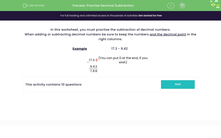# Practise Decimal Subtraction

In this worksheet, students will practise subtraction of decimals.Key stage:  KS 3

Curriculum topic:   Number

Curriculum subtopic:   Use Four Operations for All Numbers

Popular topics:   Decimals worksheets

Difficulty level:#### Worksheet Overview

In this activity, we will practise the subtraction of decimal numbers.

When adding or subtracting decimal numbers, be sure to keep the numbers and the decimal point in the right columns.

Example

17.3 - 9.42

In the calculation below, we have put 0 at the end to make it easier to see how to line up the digits correctly.

 - 1 7 . 3 0 9 . 4 2 7 . 8 8

If we fail to line the columns or decimal point up correctly, we will be mixing up our place values and it could result in some crazy answers.

Once we've set the calculation up correctly, it is just a simple subtraction problem. In the example above, we need to borrow from neighbouring columns - can you remember how to do that?

Be careful when lining up those decimal points!!### What is EdPlace?

We're your National Curriculum aligned online education content provider helping each child succeed in English, maths and science from year 1 to GCSE. With an EdPlace account you’ll be able to track and measure progress, helping each child achieve their best. We build confidence and attainment by personalising each child’s learning at a level that suits them.

Get started